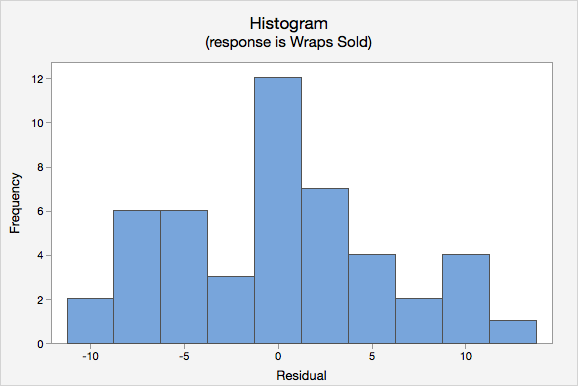# 12.3.4.2 - Example: Business Decisions

A student-run cafe wants to use data to determine how many wraps they should make today. If they make too many wraps they will have waste. But, if they don't make enough wraps they will lose out on potential profit. They have been collecting data concerning their daily sales as well as data concerning the daily temperature. They found that there is a statistically significant relationship between daily temperature and coffee sales. So, the students want to know if a similar relationship exists between daily temperature and wrap sales. The video below will walk you through the process of using simple linear regression to determine if daily temperature can be used to predict wrap sales.  The screen shots and annotation below the video will walk you through these steps again.

## Research question: Section

Can daily temperature be used to predict wrap sales?

1. Check assumptions and write hypotheses
• $$H_0: \beta_1 =0$$
• $$H_a: \beta_1 \neq 0$$

The scatterplot below shows that the relationship between maximum daily temperature and wrap sales is linear (or at least it's not non-linear). Though the relationship appears to be weak.The plot of residuals versus fits below can be used to check the assumptions of independent errors and equal error variances. There is not a significant correlation between the residuals and fits, therefore the assumption of independent errors has been met. The variance of the residuals is relatively consistent for all fitted values, therefore the assumption of equal error variances has been met.Finally, we must check for the normality of errors. We can use the normal probability plot below to check that our data points fall near the line. Or, we can use the histogram of residuals below to check that the errors are approximately normally distributed.Now that we have check all of the assumptions of simple linear regression, we can examine the regression model.

2. Calculate the test statistic
Analysis of Variance
Regression 1 16.41 16.4053 0.47 0.4961
Max Daily Temperature (F) 1 16.41 16.4053 0.47 0.4961
Error 45 1567.55 34.8345
Lack-of-Fit 24 875.17 36.4654 1.11 0.4106
Pure Error 21 692.38 32.9706
Total 46 1583.96
Model Summary
5.90208 1.04% 0.00% 0.00%
Coefficients
Term Coef SE Coef T-Value P-Value VIF
Constant 11.418 2.665 4.29 <0.0001
Max Daily Temperature (F) 0.04139 0.06032 0.69 0.4961 1.00
 Wraps Sold = 11.418 + 0.04139 Max Daily Temperature (F)

$$t = 0.69$$

3. Determine the p-value

$$p=0.4961$$

4. Make a decision

$$p > \alpha$$, fail to reject the null hypothesis

5. State a "real world" conclusion

There is not evidence that maximum daily temperature can be used to predict the number of wraps sold in the population of all days.